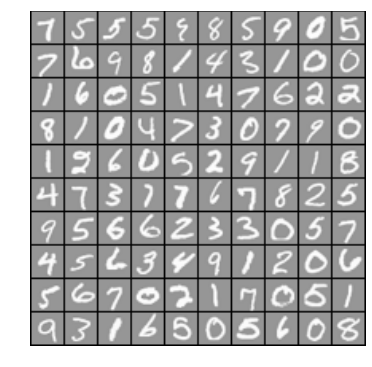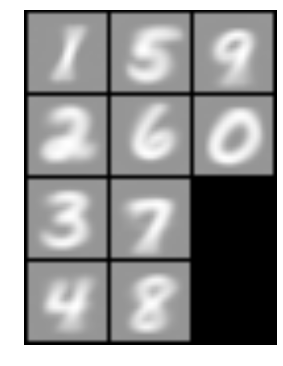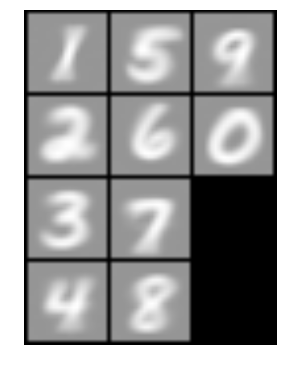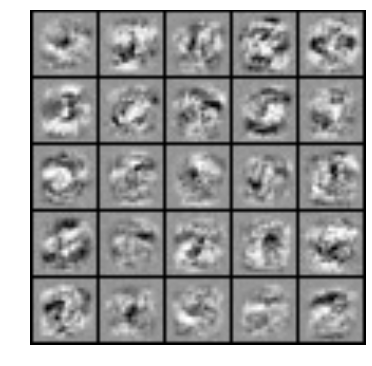## 4. Neural Networks Learning in Theano¶

The aim of this IPython notebook is to show some features of the Python Theano library in the field of machine learning. It has been developped by the LISA group at the University of Montreal (see: http://deeplearning.net/software/theano/). The notebook also relies on other standard Python libraries such as numpy, pandas and matplotlib.

To exemplify the use of Theano, this notebook solves the assignments of the Machine Learning MOOC provided by Coursera (see: https://www.coursera.org/learn/machine-learning) and performed in Stanford University by Andrew Ng (see: http://www.andrewng.org/).

The original MOOC assignments should to be programmed with the Octave language (see: https://www.gnu.org/software/octave/).The idea with this notebook is to provide Python developpers with interesting examples programmed using Theano.

This notebook has been developped using the Anaconda Python 3.4 distribution provided by Continuum Analytics (see: https://www.continuum.io/). It requires the Jupyter Notebook (see: http://jupyter.org/).

### 4.1 Neural Networks¶

#### 4.1.1 Visualizing the data¶

In :
import theano
import numpy as np
import theano.tensor as T
from theano.tensor.nnet import sigmoid
import scipy.io as sio
data = sio.loadmat('data/ex4data1.mat')
X, Y = data['X'], data['y'].T
m = X.shape

In :
%matplotlib inline
import pandas as pnd
import matplotlib.pyplot as plt

def display_data(X, Y=None):
example_width = int(np.sqrt(X.shape))

# Compute rows, cols
m, n = X.shape
example_height = int(n / example_width)

# Compute number of items to display
display_rows = int(np.floor(np.sqrt(m)))
display_cols = int(np.ceil(m / display_rows))

# Between images padding
pad = 1

# Setup blank display
display_array = - np.ones((pad + display_rows * (example_height + pad),\
pad + display_cols * (example_width + pad)))

# Copy each example into a patch on the display array
# dataset contains 20 pixel by 20 pixel grayscale images of the digit
curr_ex = 0
for j in range(display_rows):
for i in range(display_cols):
if curr_ex >= m:
break
# Get the max value of the patch
max_val = max(abs(X[curr_ex]))
display_array[(pad + j * (example_height + pad)):(pad + j * (example_height + pad))+example_height,\
(pad + i * (example_width + pad)):(pad + i * (example_width + pad))+example_width] =\
np.reshape(X[curr_ex], (example_height, example_width)) / max_val
curr_ex += 1
if curr_ex >= m:
break

plt.figure(figsize=(8,6))
plt.axis('off')
plt.imshow(display_array.T, cmap="Greys_r")

if Y is not None:
df = pnd.DataFrame(Y.reshape((display_rows, display_cols)).T)
df = df.replace(10,0) # 0 is mapped to 10 in Y
print(df)

In :
rnd = np.random.permutation(m)[0:100]
sel = X[rnd,:] # 100 random digits
res = Y[rnd]
display_data(sel, res)

   0  1  2  3  4  5  6  7  8  9
0  7  5  5  5  9  8  5  9  0  5
1  7  6  9  8  1  4  3  1  0  0
2  1  6  0  5  1  4  7  6  2  2
3  8  1  0  4  7  3  0  7  9  0
4  1  2  6  0  5  2  9  1  1  8
5  4  7  3  7  7  6  7  8  2  5
6  9  5  6  6  2  3  3  0  5  7
7  4  5  6  3  4  9  1  2  0  6
8  5  6  7  0  2  1  7  0  5  1
9  9  3  1  6  5  0  5  6  0  8#### 4.1.2 Feedforward and cost function¶

In :
def T_add_ones(x, a, b):
var = T.concatenate([T.ones(a), x.flatten().T])
return var.reshape((b, a))

X1 = np.c_[np.ones(m), X] # Add intercept term to X
X1 = X1.T

# load precomputed network parameters
weights = sio.loadmat('data/ex4weights.mat')
t1 = weights['Theta1']
t2 = weights['Theta2']

print("X1:", X1.shape)
print("theta1:", t1.shape)
print("theta2:", t2.shape)

# layer sizes
input_layer_size = X.shape
hidden_layer_size = t1.shape
num_labels = t2.shape

print('\ninput_layer_size: %i' % input_layer_size)
print('hidden_layer_size: %i' % hidden_layer_size)
print('num_labels: %i' % num_labels)

x = T.matrix('x')
y = T.vector('y')
theta1 = theano.shared(t1, 'theta1')
theta2 = theano.shared(t2, 'theta2')

# first layer
y1 = sigmoid(T.dot(theta1, x))

# second layer
y2 = T_add_ones(y1, m, hidden_layer_size+1)
y3 = sigmoid(T.dot(theta2, y2))

J = 0
for i in range(num_labels):
yy = T.eq(y,i+1)
J += -T.sum(yy * T.log(y3[i,:]) + (1 - yy) * T.log(1 - y3[i,:])) / m

cost = theano.function([x, y], J)

print('\ncost: %f' % cost(X1, Y))

X1: (401, 5000)
theta1: (25, 401)
theta2: (10, 26)

input_layer_size: 400
hidden_layer_size: 25
num_labels: 10

cost: 0.287629


#### 4.1.3 Regularized cost function¶

In :
# Weight regularization parameter (we set this to 0 here).
lambda_ = 1

x = T.matrix('x')
y = T.vector('y')
theta1 = theano.shared(t1, 'theta1')
theta2 = theano.shared(t2, 'theta2')

# first layer
y1 = sigmoid(T.dot(theta1, x))

# second layer
y2 = T_add_ones(y1, m, hidden_layer_size+1)
y3 = sigmoid(T.dot(theta2, y2))

# regularization on theta1 and theta2
reg = lambda_ * (T.sum(theta1[:,1:]**2) + T.sum(theta2[:,1:]**2)) / 2 / m
J = reg
for i in range(num_labels):
yy = T.eq(y,i+1)
J += -T.sum(yy * T.log(y3[i,:]) + (1 - yy) * T.log(1 - y3[i,:])) / m

cost = theano.function([x, y], J)

print('cost: %f' % cost(X1, Y))

cost: 0.383770


## 4.2 Backpropagation¶

### 4.2.1 Sigmoid gradient¶

In :
x = T.matrix('x')
h = 1.0 / (1.0 + T.exp(-x))
h_grad = h * (1-h)

sigmoid2 = theano.function([x], h)
sigmoid2_grad = theano.function([x], h*(1-h))
print(sigmoid2(np.array([[-1, -0.5, 0, 0.5, 1]])))
print(sigmoid2_grad(np.array([[-1, -0.5, 0, 0.5, 1]])))

[[ 0.26894142  0.37754067  0.5         0.62245933  0.73105858]]
[[ 0.19661193  0.23500371  0.25        0.23500371  0.19661193]]


### 4.2.2 Random initialization¶

In :
def rand_initialize_weights(l_in, l_out, epsilon_init):
return np.random.rand(l_out, l_in+1) * 2 * epsilon_init - epsilon_init

epsilon_init = 0.12
t1 = rand_initialize_weights(input_layer_size, hidden_layer_size, epsilon_init)
t2 = rand_initialize_weights(hidden_layer_size, num_labels, epsilon_init)


### 4.2.3 Backpropagation¶

In :
def T_add_ones(x, a, b):
var = T.concatenate([T.ones(a), x.flatten().T])
return var.reshape((b, a))

theta1 = theano.shared(t1, 'theta1')
theta2 = theano.shared(t2, 'theta2')

# Choose some alpha value
alpha = 1

x = T.matrix('x')
y = T.vector('y')

# first layer
y1 = sigmoid(T.dot(theta1, x))

# second layer
y2 = T_add_ones(y1, m, hidden_layer_size+1)
y3 = sigmoid(T.dot(theta2, y2))

J = 0
for i in range(num_labels):
yy = T.eq(y,i+1)
J += -T.sum(yy * T.log(y3[i,:]) + (1 - yy) * T.log(1 - y3[i,:])) / m

grad1, grad2 = T.grad(J, [theta1, theta2])

train = theano.function([x,y], [J, y3], updates = [(theta1, theta1-alpha*grad1), (theta2, theta2-alpha*grad2)])

for i in range(2000):
costM, y3 = train(X1,Y)
if i%500 == 0:
print('[%i] %f' % (i, costM))

print('cost: %f' % costM)

 6.759750
 0.481384
 0.335062
 0.257458
cost: 0.206653

In :
# number of positive predictions where best prediction == Y-1
accuracy = np.sum(np.argmax(y3, axis=0) == (Y-1))/len(Y)
print('Train Accuracy: %f' % accuracy)

Train Accuracy: 0.980200


### 4.2.4 Regularized Neural Networks¶

In :
theta1 = theano.shared(t1, 'theta1')
theta2 = theano.shared(t2, 'theta2')

# Weight regularization parameter (we set this to 0 here).
lambda_ = 3

# Choose some alpha value
alpha = 1

x = T.matrix('x')
y = T.vector('y')

# first layer
y1 = sigmoid(T.dot(theta1,x))

# second layer
y2 = T_add_ones(y1, m, hidden_layer_size+1)
y3 = sigmoid(T.dot(theta2, y2))

# regularization on theta1 and theta2
reg = lambda_ * (T.sum(theta1[:,1:]**2) + T.sum(theta2[:,1:]**2)) / 2 / m

J = reg
for i in range(num_labels):
yy = T.eq(y,i+1)
J += -T.sum(yy * T.log(y3[i,:]) + (1 - yy) * T.log(1 - y3[i,:])) / m

grad1, grad2 = T.grad(J, [theta1, theta2])

train = theano.function([x,y], [J, y3, theta1], updates = [(theta1, theta1-alpha*grad1), (theta2, theta2-alpha*grad2)])

for i in range(2000):
costM, y3, theta1 = train(X1,Y)
if i%500 == 0:
print('[%i] %f' % (i, costM))

print('cost: %f' % costM)

 6.774405
 0.711646
 0.645442
 0.620571
cost: 0.607821

In :
# number of positive predictions where best prediction == Y-1
accuracy = np.sum(np.argmax(y3, axis=0) == (Y-1))/len(Y)
print('Train Accuracy: %f' % accuracy)

Train Accuracy: 0.963000


### 4.2.5 Mean Tagged and Recognized Digit Set¶

In :
# display mean tagged digit set
z1 = np.concatenate([np.mean(X[Y == i+1], axis=0) for i in range(10)])
display_data(z1.reshape((10,400)))In :
# display mean recognized digit set
z2 = np.concatenate([np.mean(X[np.argmax(y3, axis=0) == i], axis=0) for i in range(10)])
display_data(z2.reshape((10,400)))In :
# z1 and z2 are quite different
np.sum(z1!=z2)

Out:
3576

## 4.3 Visualizing the hidden layer¶

In :
# take theta1 without the bias term
z = theta1[:,1:]
display_data(z)In [ ]: